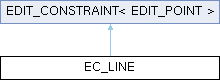KiCad PCB EDA Suite
EC_LINE Class Reference

EDIT_CONSTRAINT that imposes a constraint that a point has to lie on a line (determined by 2 points). More...

`#include <edit_constraints.h>`

Inheritance diagram for EC_LINE:## Public Member Functions

EC_LINE (EDIT_POINT &aConstrained, const EDIT_POINT &aConstrainer)
Correct coordinates of the constrained edit handle. More...

virtual void Apply (EDIT_POINT &aHandle) override
Correct coordinates of the constrained edit handle. More...

void Apply ()
Correct coordinates of the constrained edit handle. More...

## Protected Attributes

EDIT_POINTm_constrained
Point that is constrained by rules implemented by Apply(). More...

## Private Attributes

const EDIT_POINTm_constrainer
Point that imposes the constraint. More...

VECTOR2I m_line
Vector representing the constraining line. More...

## Detailed Description

EDIT_CONSTRAINT that imposes a constraint that a point has to lie on a line (determined by 2 points).

Definition at line 152 of file edit_constraints.h.

## ◆ EC_LINE()

 EC_LINE::EC_LINE ( EDIT_POINT & aConstrained, const EDIT_POINT & aConstrainer )

Correct coordinates of the constrained edit handle.

Definition at line 63 of file edit_constraints.cpp.

63  :
64  EDIT_CONSTRAINT<EDIT_POINT>( aConstrained ), m_constrainer( aConstrainer )
65 {
67 }
VECTOR2I m_line
Vector representing the constraining line.
virtual VECTOR2I GetPosition() const
Return coordinates of an EDIT_POINT.
Definition: edit_points.h:70
EDIT_POINT & m_constrained
Point that is constrained by rules implemented by Apply().
const EDIT_POINT & m_constrainer
Point that imposes the constraint.

## ◆ Apply() [1/2]

 void EDIT_CONSTRAINT< EDIT_POINT >::Apply ( )
inlineinherited

Correct coordinates of the constrained edit handle.

Definition at line 71 of file edit_constraints.h.

72  {
74  }
void Apply()
Correct coordinates of the constrained edit handle.
EDIT_POINT & m_constrained
Point that is constrained by rules implemented by Apply().

## ◆ Apply() [2/2]

 void EC_LINE::Apply ( EDIT_POINT & aHandle )
overridevirtual

Correct coordinates of the constrained edit handle.

Implements EDIT_CONSTRAINT< EDIT_POINT >.

Definition at line 70 of file edit_constraints.cpp.

71 {
73  SEG projection( aHandle.GetPosition(), aHandle.GetPosition() + m_line.Perpendicular() );
74
75  if( OPT_VECTOR2I intersect = projection.IntersectLines( main ) )
76  aHandle.SetPosition( *intersect );
77 }
VECTOR2I m_line
Vector representing the constraining line.
main()
VECTOR2< T > Perpendicular() const
Compute the perpendicular vector.
Definition: vector2d.h:314
static bool intersect(const SEGMENT_WITH_NORMALS &aSeg, const SFVEC2F &aStart, const SFVEC2F &aEnd)
Definition: polygon_2d.cpp:273
virtual VECTOR2I GetPosition() const
Return coordinates of an EDIT_POINT.
Definition: edit_points.h:70
OPT< VECTOR2I > OPT_VECTOR2I
Definition: seg.h:38
Definition: seg.h:40
const EDIT_POINT & m_constrainer
Point that imposes the constraint.
virtual void SetPosition(const VECTOR2I &aPosition)
Set new coordinates for an EDIT_POINT.
Definition: edit_points.h:106

## ◆ m_constrained

 EDIT_POINT & EDIT_CONSTRAINT< EDIT_POINT >::m_constrained
protectedinherited

Point that is constrained by rules implemented by Apply().

Definition at line 77 of file edit_constraints.h.

## ◆ m_constrainer

 const EDIT_POINT& EC_LINE::m_constrainer
private

Point that imposes the constraint.

Definition at line 161 of file edit_constraints.h.

Referenced by Apply(), and EC_LINE().

## ◆ m_line

 VECTOR2I EC_LINE::m_line
private

Vector representing the constraining line.

Definition at line 162 of file edit_constraints.h.

Referenced by Apply(), and EC_LINE().

The documentation for this class was generated from the following files: